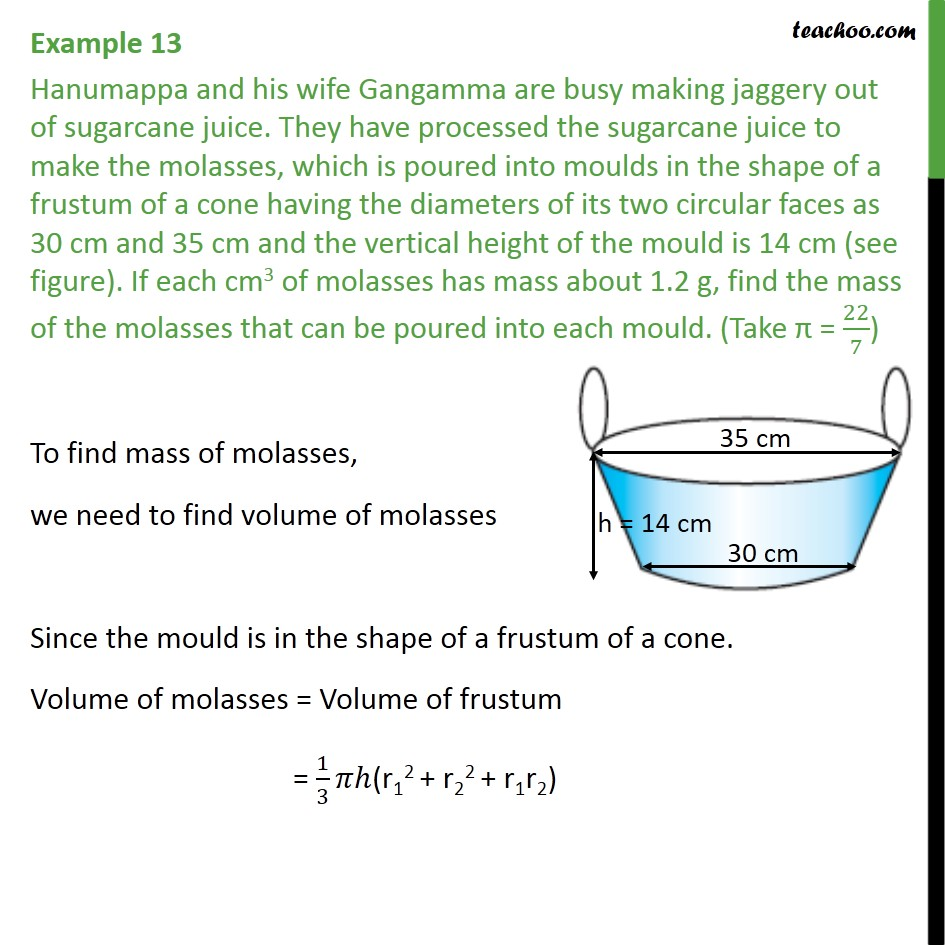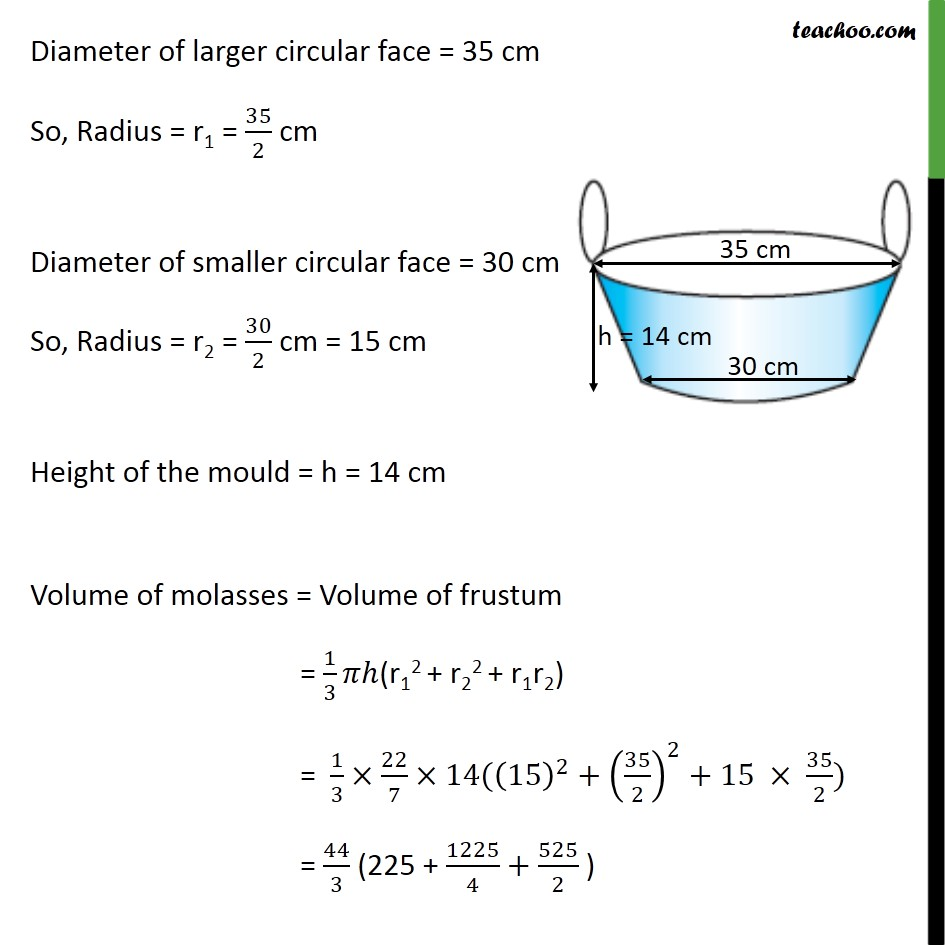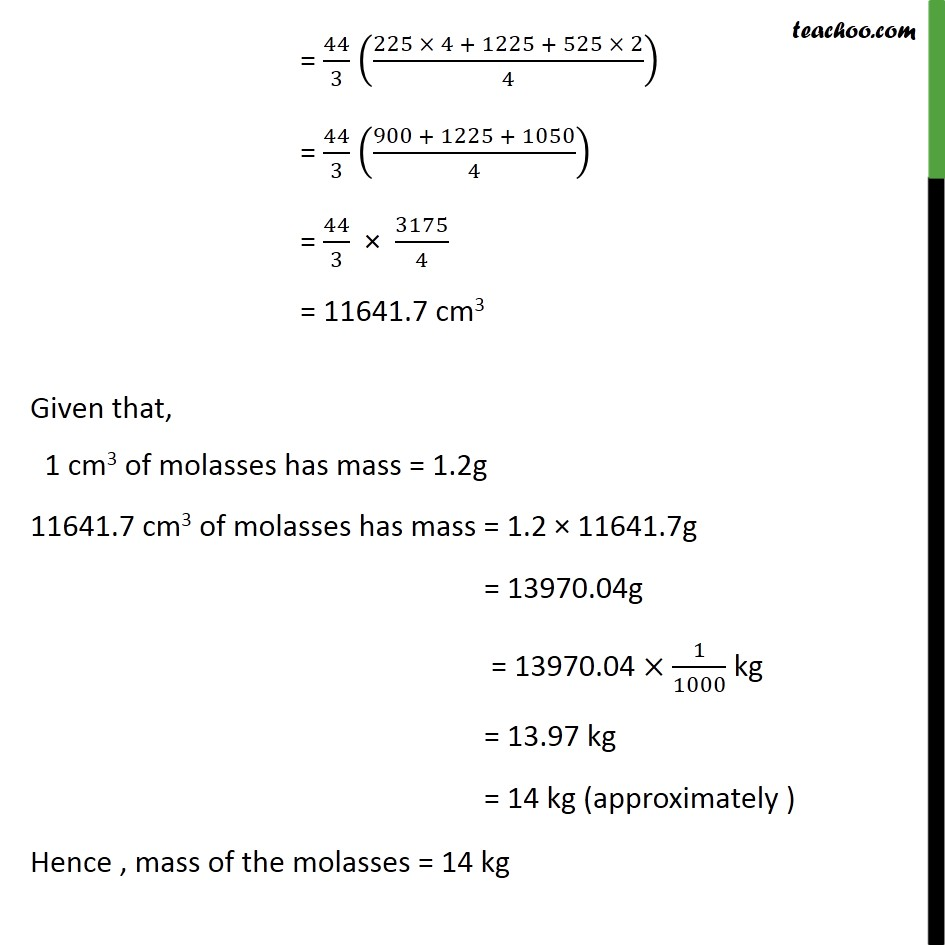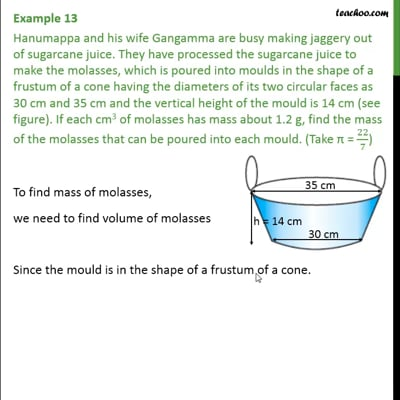Examples

Chapter 13 Class 10 Surface Areas and Volumes
Serial order wiseThis video is only available for Teachoo black users

Solve all your doubts with Teachoo Black (new monthly pack available now!)

### Transcript

Example 13 Hanumappa and his wife Gangamma are busy making jaggery out of sugarcane juice. They have processed the sugarcane juice to make the molasses, which is poured into moulds in the shape of a frustum of a cone having the diameters of its two circular faces as 30 cm and 35 cm and the vertical height of the mould is 14 cm (see figure). If each cm3 of molasses has mass about 1.2 g, find the mass of the molasses that can be poured into each mould. (Take = 22/7) To find mass of molasses, we need to find volume of molasses Since the mould is in the shape of a frustum of a cone. Volume of molasses = Volume of frustum = 1/3 (r12 + r22 + r1r2) Diameter of larger circular face = 35 cm So, Radius = r1 = 35/2 cm Diameter of smaller circular face = 30 cm So, Radius = r2 = 30/2 cm = 15 cm Height of the mould = h = 14 cm Volume of molasses = Volume of frustum = 1/3 (r12 + r22 + r1r2) = 1/3 22/7 14((15)^2+(35/2)^2+15 35/2) = 44/3 (225 + 1225/4+525/2 ) = 44/3 ((225 4 + 1225 + 525 2)/4) = 44/3 ((900 + 1225 + 1050)/4) = 44/3 3175/4 = 11641.7 cm3 Given that, 1 cm3 of molasses has mass = 1.2g 11641.7 cm3 of molasses has mass = 1.2 11641.7g = 13970.04g = 13970.04 1/1000 kg = 13.97 kg = 14 kg (approximately ) Hence , mass of the molasses = 14 kg